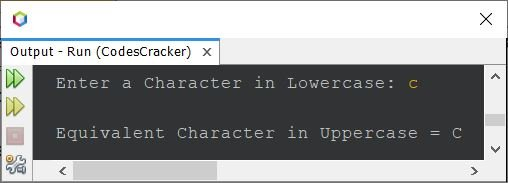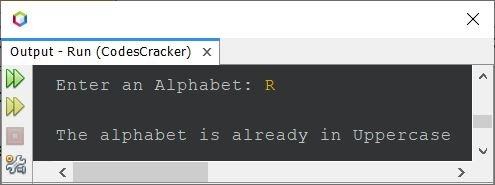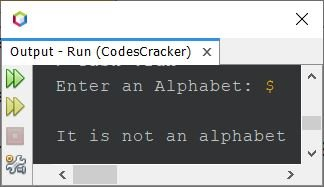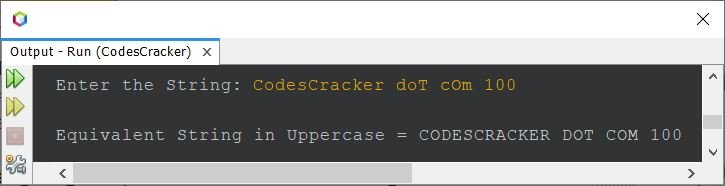# Java Program to Convert Lowercase to Uppercase

This post covers a program in Java that converts lowercase character or string to uppercase character or string with and without using string function. To achieve this task, either we can use the ASCII values or a string function named toUpperCase().

## Lowercase Character to Uppercase in Java using ASCII

The question is, write a Java program that converts a lowercase character to uppercase using ASCII value. The character must be received by user at run-time of the program. The program given below is its answer:

The ASCII value of 'A' is 65 and 'a' is 97. That is, 97-65 = 32, indicates that the ASCII value of lowercase character is 32 more than the ASCII value of equivalent uppercase character. So to convert character from lowercase to uppercase character, just subtract 32 using ASCII, as shown in the program given below.

```import java.util.Scanner;

public class CodesCracker
{
public static void main(String[] args)
{
char ch;
int ascii;
Scanner scan = new Scanner(System.in);

System.out.print("Enter a Character in Lowercase: ");
ch = scan.next().charAt(0);

ascii = ch;
ascii = ascii - 32;
ch = (char)ascii;

System.out.println("\nEquivalent Character in Uppercase = " +ch);
}
}```

The snapshot given below shows the sample run of above Java program, with user input c as character to convert it in uppercase:Since the ASCII values of A-Z are 65-90. Whereas the ASCII values of a-z are 97-122. Therefore as already told, there is a difference of 32 between A and a, or B and b, and so on. That is, to convert from a to A, we need to subtract 32.

In above program, the statement:

`ascii = ch;`

indicates that the value of ch gets initialized to ascii. And because ascii is of int type, therefore instead of initializing the character itself, its ASCII value gets initialized. That is, ascii value (99) of 'c' will get initialized to ascii. Now using the statement:

`ascii = ascii - 32;`

99-32 or 67 will get initialized to ascii. Again using the statement:

`ch = (char)ascii;`

In above statement, the code (char)67 evaluated into the character corresponding to ASCII value 67, that is C will get initialized to ch.

Note - I've used char to convert ASCII value to its equivalent character, before initializing to a char-type variable, to avoid loosly conversion from int to char. But in case of char to int, we need not to covert manually, because of the types promotion rules.

The above program can also be created in this way:

```import java.util.Scanner;

public class CodesCracker
{
public static void main(String[] args)
{
Scanner scan = new Scanner(System.in);

System.out.print("Enter a Character in Lowercase: ");
char ch = scan.next().charAt(0);

System.out.println("\nEquivalent Character in Uppercase = " +(char)(ch-32));
}
}```

You'll get the same output as of previous program.

## Lowercase Character to Uppercase in Java - Complete Version

Since the program given above has a limitation. That limitation is, when user enters a character already in uppercase, then the program in that case too, do the process of getting its ASCII value and subtracting by 32, then printing whatever the result comes out, as equivalent character in uppercase, that looks weird, also not a correct program. Therefore the program needs to be modified. Here is the modified version of the above program:

```import java.util.Scanner;

public class CodesCracker
{
public static void main(String[] args)
{
Scanner scan = new Scanner(System.in);

System.out.print("Enter an Alphabet: ");
char ch = scan.next().charAt(0);

int ascii = ch;
if(ascii>=97 && ascii<=122)
{
ascii = ascii - 32;
ch = (char)ascii;
System.out.println("\nEquivalent Character in Uppercase = " +ch);
}
else if(ascii>=65 && ascii<=90)
System.out.println("\nThe alphabet is already in Uppercase");
else
System.out.println("\nIt is not an alphabet");

}
}```

The sample run of above program with user input R:Here is another sample run with user input \$ (not an alphabet input):## Lowercase String to Uppercase in Java without using String Function

This program converts lowercase string to uppercase string without using the string function toUpperCase().

```import java.util.Scanner;

public class CodesCracker
{
public static void main(String[] args)
{
String str;
int len, i, ascii;
char ch;
Scanner scan = new Scanner(System.in);

System.out.print("Enter the String: ");
str = scan.nextLine();

len = str.length();
char[] strChars = new char[len];
char[] strUpperChars = new char[len];
for(i=0; i<len; i++)
strChars[i] = str.charAt(i);

for(i=0; i<len; i++)
{
ch = strChars[i];
ascii = ch;
if(ascii>=97 && ascii<=122)
{
ascii = ascii - 32;
ch = (char)ascii;
strUpperChars[i] = ch;
}
else
strUpperChars[i] = ch;
}

System.out.print("\nString in Uppercase: ");
for(i=0; i<len; i++)
System.out.print(strUpperChars[i]);
}
}```

Here is its sample run with user input cOdesCRAcker dOt cOm @098 as string. I've supplied both lowercase and uppercase alphabet along with some other characters:In above program, the entered string stored in str, gets initialized to a character array strChars in character-by-character manner. And further, each and every lowercase alphabet of this character array gets capitalized, and initialized to another character array named strUpperChars. Finally, I've printed the value of strUpperChars, character-by-character.

## Lowercase String to Uppercase in Java using String Function

This is the last program of this post, created using a string function to convert lowercase string to uppercase directly.

```import java.util.Scanner;

public class CodesCracker
{
public static void main(String[] args)
{
String str;
Scanner scan = new Scanner(System.in);

System.out.print("Enter the String: ");
str = scan.nextLine();

str = str.toUpperCase();
System.out.println("\nEquivalent String in Uppercase = " +str);
}
}```

The sample run of above program with user input CodesCracker doT cOm 100 as string:#### Same Program in Other Languages

Java Online Test

« Previous Program Next Program »